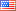Revision history [back]

Matrix Multiplication

I am trying to multiply the following:

Iz=1/2matrix(2,2,[[1,0],[0,-1]]) a=(1,0) Iza

This should work, what am I doing wrong? I would expect (1/2, 0)

Sage returns the following error:
Traceback (click to the left of this block for traceback) ... TypeError: 'sage.matrix.matrix_rational_dense.Matrix_rational_dense' object cannot be interpreted as an index

Traceback (most recent call last): File "<stdin>", line 1, in <module> File "_sage_input_149.py", line 10, in <module> exec compile(u'open("___code___.py","w").write("# -- coding: utf-8 --\n" + _support_.preparse_worksheet_cell(base64.b64decode("SXo9MS8yKm1hdHJpeCgyLDIsW1sxLDBdLFswLC0xXV0pO0l6CmE9KDEsMCkKSXoqYQ=="),globals())+"\n"); execfile(os.path.abspath("___code___.py")) File "", line 1, in <module>

File "/tmp/tmpJoBb2Y/___code___.py", line 5, in <module> exec compile(u'Iz*a File "", line 1, in <module>

File "element.pyx", line 2260, in sage.structure.element.Matrix.__mul__ (sage/structure/element.c:14881) File "coerce.pyx", line 759, in sage.structure.coerce.CoercionModel_cache_maps.bin_op (sage/structure/coerce.c:6940) TypeError: 'sage.matrix.matrix_rational_dense.Matrix_rational_dense' object cannot be interpreted as an index

Thanx2 No.2 RevisionMike Hansen4038 ●23 ●48 ●89

Matrix Multiplication

I am trying to multiply the following:

Iz=1/2matrix(2,2,[[1,0],[0,-1]])

Iz=1/2*matrix(2,2,[[1,0],[0,-1]])
a=(1,0)
Iza

Iz*a

This should work, what am I doing wrong? I would expect (1/2, 0)

Sage returns the following error:

Traceback (click to the left of this block for traceback)
...
TypeError: 'sage.matrix.matrix_rational_dense.Matrix_rational_dense'
object cannot be interpreted as an index

index

Traceback (most recent call last): File "<stdin>", line 1, in <module> File "_sage_input_149.py", line 10, in <module> exec compile(u'open("___code___.py","w").write("# -- -*- coding: utf-8 --\n" -*-\\n" + _support_.preparse_worksheet_cell(base64.b64decode("SXo9MS8yKm1hdHJpeCgyLDIsW1sxLDBdLFswLC0xXV0pO0l6CmE9KDEsMCkKSXoqYQ=="),globals())+"\n"); _support_.preparse_worksheet_cell(base64.b64decode("SXo9MS8yKm1hdHJpeCgyLDIsW1sxLDBdLFswLC0xXV0pO0l6CmE9KDEsMCkKSXoqYQ=="),globals())+"\\n"); execfile(os.path.abspath("___code___.py")) File "", line 1, in <module>

<module>

File "/tmp/tmpJoBb2Y/___code___.py", line 5, in <module> exec compile(u'Iz*a File "", line 1, in <module>

<module>

File "element.pyx", line 2260, in sage.structure.element.Matrix.__mul__ (sage/structure/element.c:14881) File "coerce.pyx", line 759, in sage.structure.coerce.CoercionModel_cache_maps.bin_op (sage/structure/coerce.c:6940) TypeError: 'sage.matrix.matrix_rational_dense.Matrix_rational_dense' object cannot be interpreted as an index

index

Thanx